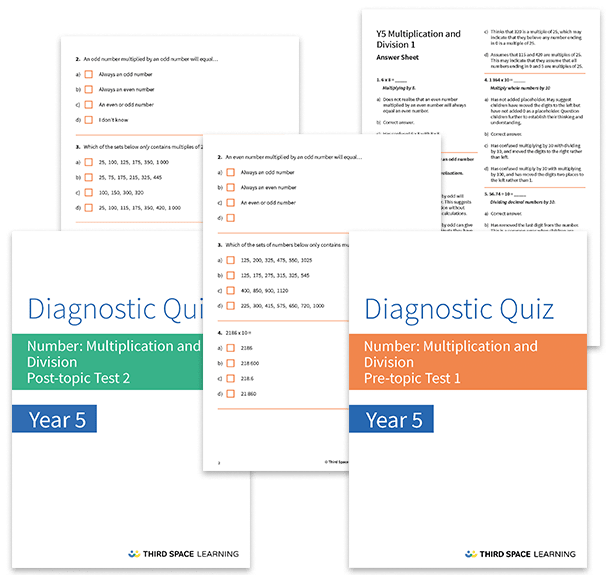# Multiplication and Division Diagnostic Assessments Year 5

This pack of resources contains two diagnostic assessments for multiplication and division, one pre and one post topic assessment. Each test contains 25 or 26 multiple choice questions with detailed answers that not only indicate the correct answer but also explain the distractor answers. You can use these tests to help you diagnose specific misconceptions and learning gaps before starting a block of learning and after the block has been completed.

These tests cover:

• Times table facts (multiplication and division) for the 1 to 12 times tables
• Identifying multiples and factors
• Multiplying and dividing by 10, 100 and 1,000 (including decimals)
• Square and cube numbers
• Multiplying by 0
• Multiplying three one-digit numbers
• Multiplying/ dividing a three-digit or four-digit numbers by a one-digit number
• Multiplying two, two-digit numbers and multiplying a three-digit number by a two-digit number

Year 5

Multiplication, Division